### 一、环境搭建

requirements.txt如下

``````numpy
matplotlib
pandas
jieba
Cython
word2vec
tensorflow
gensim
scikit-learn
python-dotenv
requests
keras
BeautifulSoup4
html5lib``````

python依赖装好之后，简单整一下cuda，其实这块TensorFlow官网GPU支持文档已经非常详细了，按着一步一步走就行了。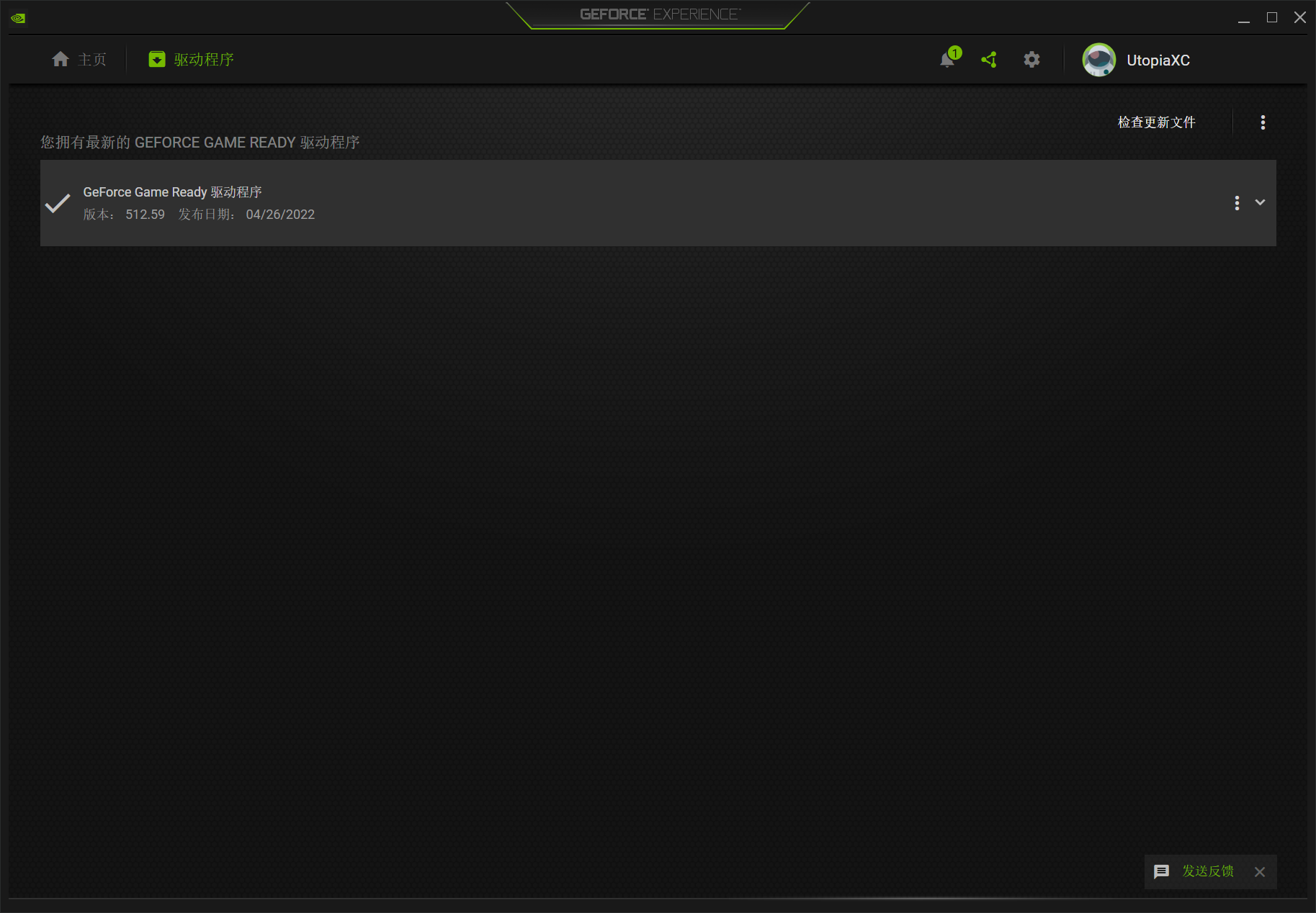``nvidia-smi``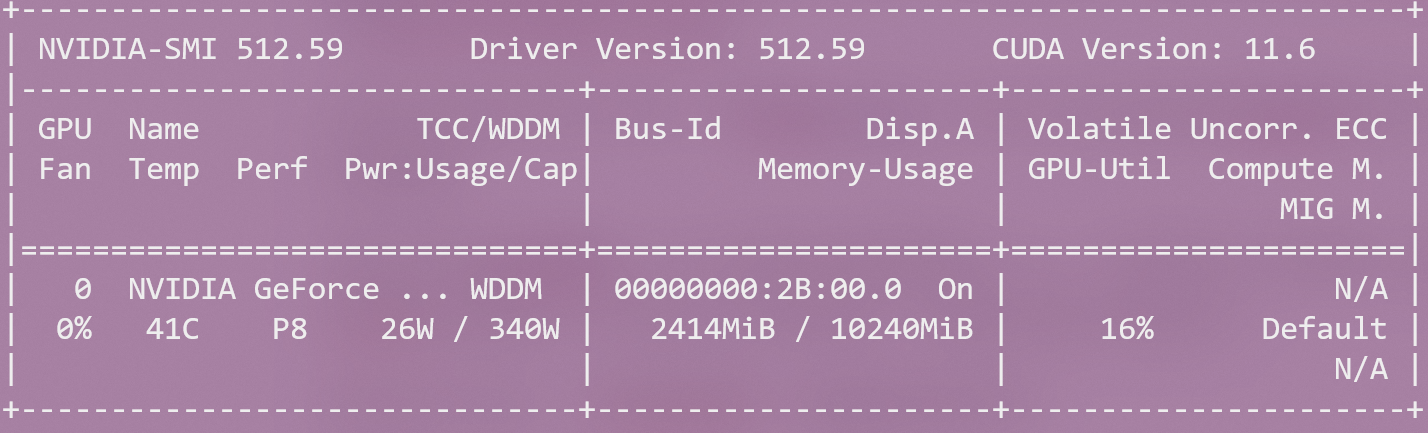### 二、数据集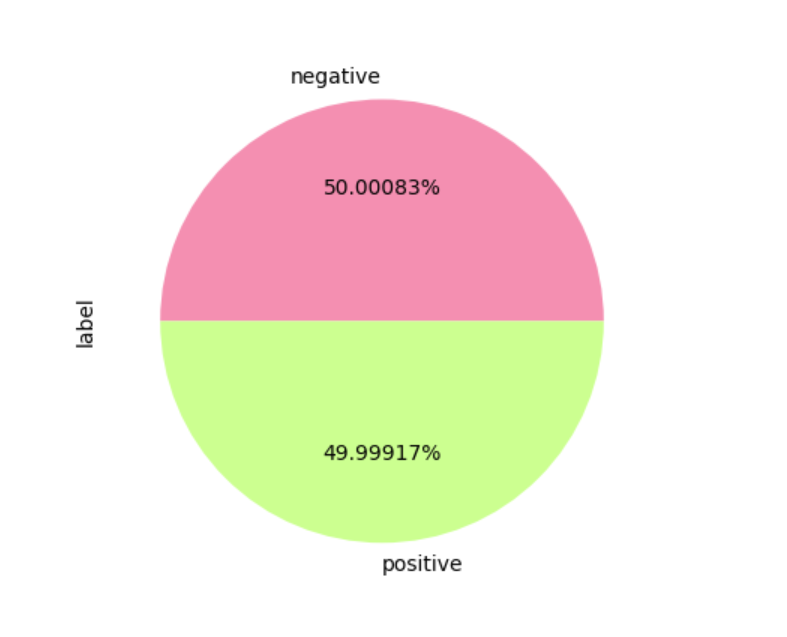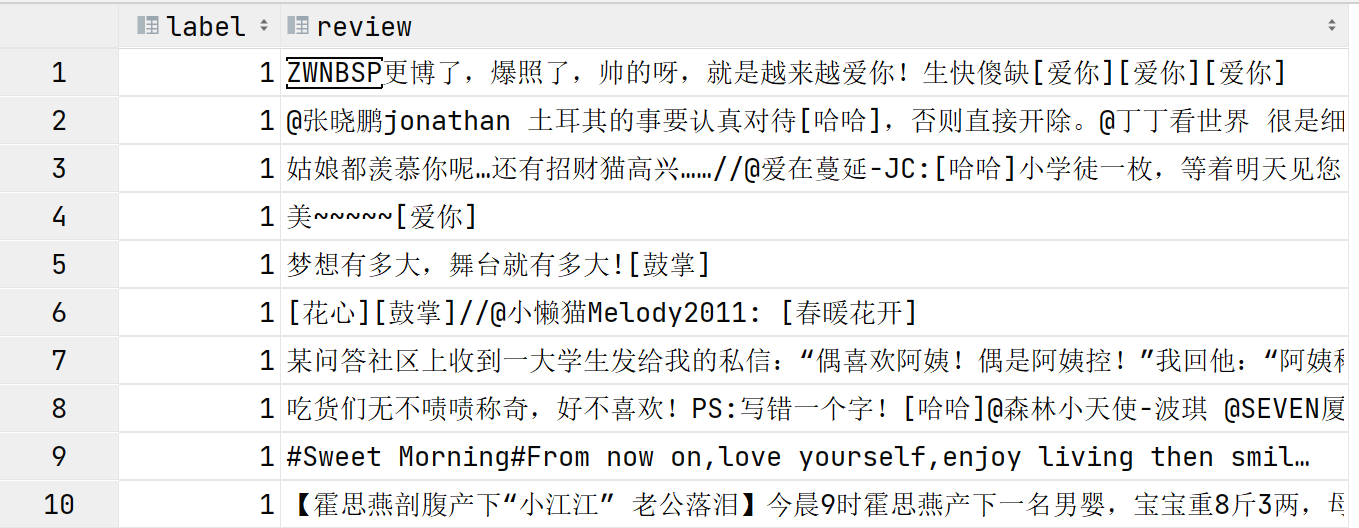``````# 引入轮子
import jieba
import matplotlib.pyplot as plt
import numpy as np
import pandas as pd
from gensim.models.word2vec import Word2Vec
from sklearn.model_selection import train_test_split
from keras.models import Sequential
from keras.layers import Dense, LSTM, Embedding

# panda加载数据

# 利用plot绘制数据集分布
df.label.value_counts().plot(kind='pie', autopct='%0.05f%%', labels=['negative', 'positive'],
colors=['#f48fb1', '#ccff90'])
plt.savefig("out/data.png")
plt.show()
plt.clf()

# 对数据集进行jieba分词
df = df.sample(frac=1)
word_cut = lambda x: jieba.lcut(x)
df['words'] = df["review"].apply(word_cut)
x = df["words"]``````

### 三、浅层网络构建

``````# word2vec模型
w2v = Word2Vec(vector_size=300,min_count=10)
w2v.build_vocab(x)
w2v.train(x,
total_examples=w2v.corpus_count,
epochs=20)

# 保存模型
w2v.save('model/w2v_model.pkl')

# 将文本转化为向量的方法
def average_vec(text):
vec = np.zeros(300).reshape((1, 300))
for word in text:
try:
vec += w2v.wv[word].reshape((1, 300))
except KeyError:
continue
return vec

# 将词向量保存为 Ndarray
x_vec = np.concatenate([average_vec(z) for z in x])
y = pd.get_dummies(df['label']).values``````

``X_train, X_test, y_train, y_test = train_test_split(x_vec, y, test_size=0.2)``

### 四、LSTM网络构建

``````model = Sequential()
model.summary()``````

### 五、模型训练与保存

``````# 训练参数
epochs = 40
batch_size = 128

# 模型训练
history = model.fit(X_train,
y_train,
epochs=epochs,
batch_size=batch_size,
validation_split=0.2)
history_dict = history.history
history_dict.keys()

#利用plot绘制损失与准确率
acc = history_dict['accuracy']
val_acc = history_dict['val_accuracy']
loss = history_dict['loss']
val_loss = history_dict['val_loss']

epochs = range(1, len(acc) + 1)

plt.plot(epochs, loss, 'bo', label='Training loss')
plt.plot(epochs, val_loss, 'b', label='Validation loss')
plt.title('Training and validation loss')
plt.xlabel('Epochs')
plt.ylabel('Loss')
plt.legend()
plt.savefig("out/loss.png")
plt.show()
plt.clf()

plt.plot(epochs, acc, 'bo', label='Training acc')
plt.plot(epochs, val_acc, 'b', label='Validation acc')
plt.title('Training and validation accuracy')
plt.xlabel('Epochs')
plt.ylabel('Accuracy')
plt.savefig("out/accuracy.png")
plt.legend()
plt.show()

# 进行一个模型的保存
model.save("model/svm_model")``````

### 六、验证结果与测试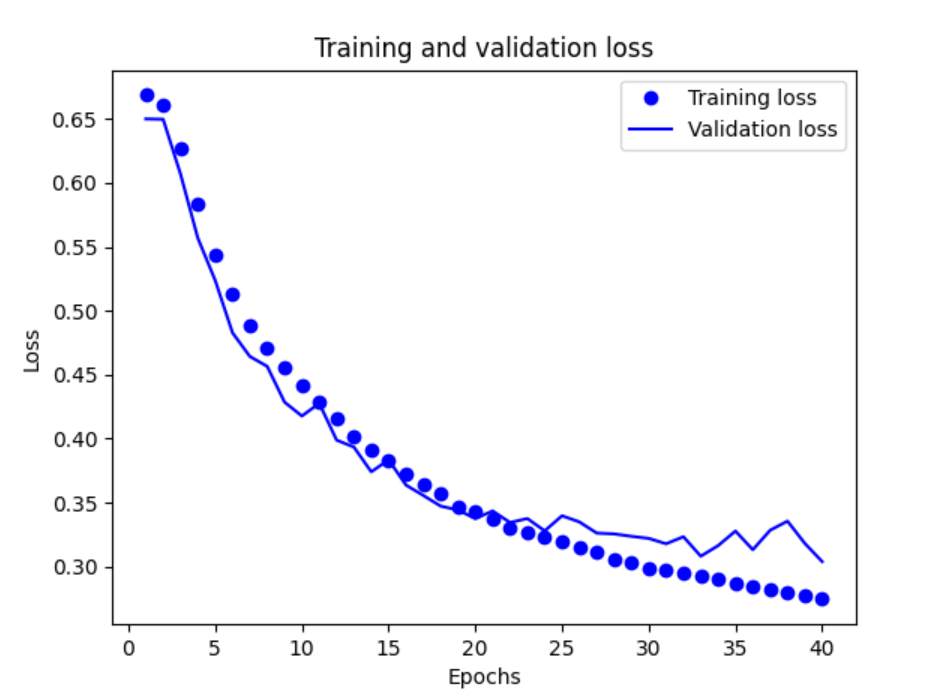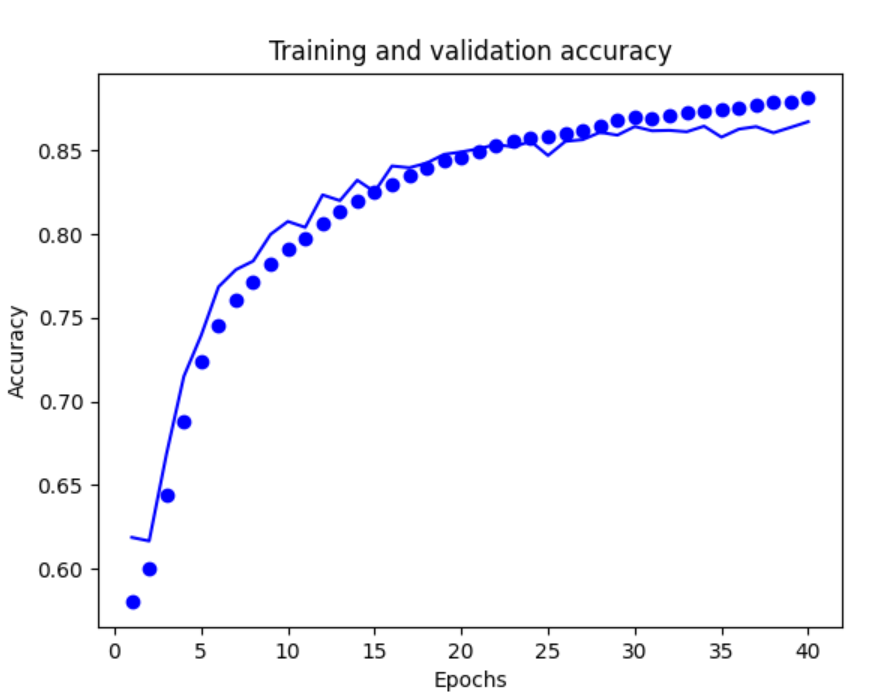``````import jieba
import numpy as np
import keras.models
from gensim.models.word2vec import Word2Vec

def average_vec(words):
vec = np.zeros(300).reshape((1, 300))
for word in words:
try:
vec += w2v.wv[word].reshape((1, 300))
except KeyError:
continue
return vec

def model_predict(string):
words = jieba.lcut(str(string))
words_vec = average_vec(words)
keras.models.logging.set_verbosity(keras.models.logging.ERROR)
result = np.argmax(model.predict(words_vec))
if int(result) == 1:
return "正向"
else:
return "负向"

while True:
text = input("input> ")
res = model_predict(text)
print(text + " | " + res)
``````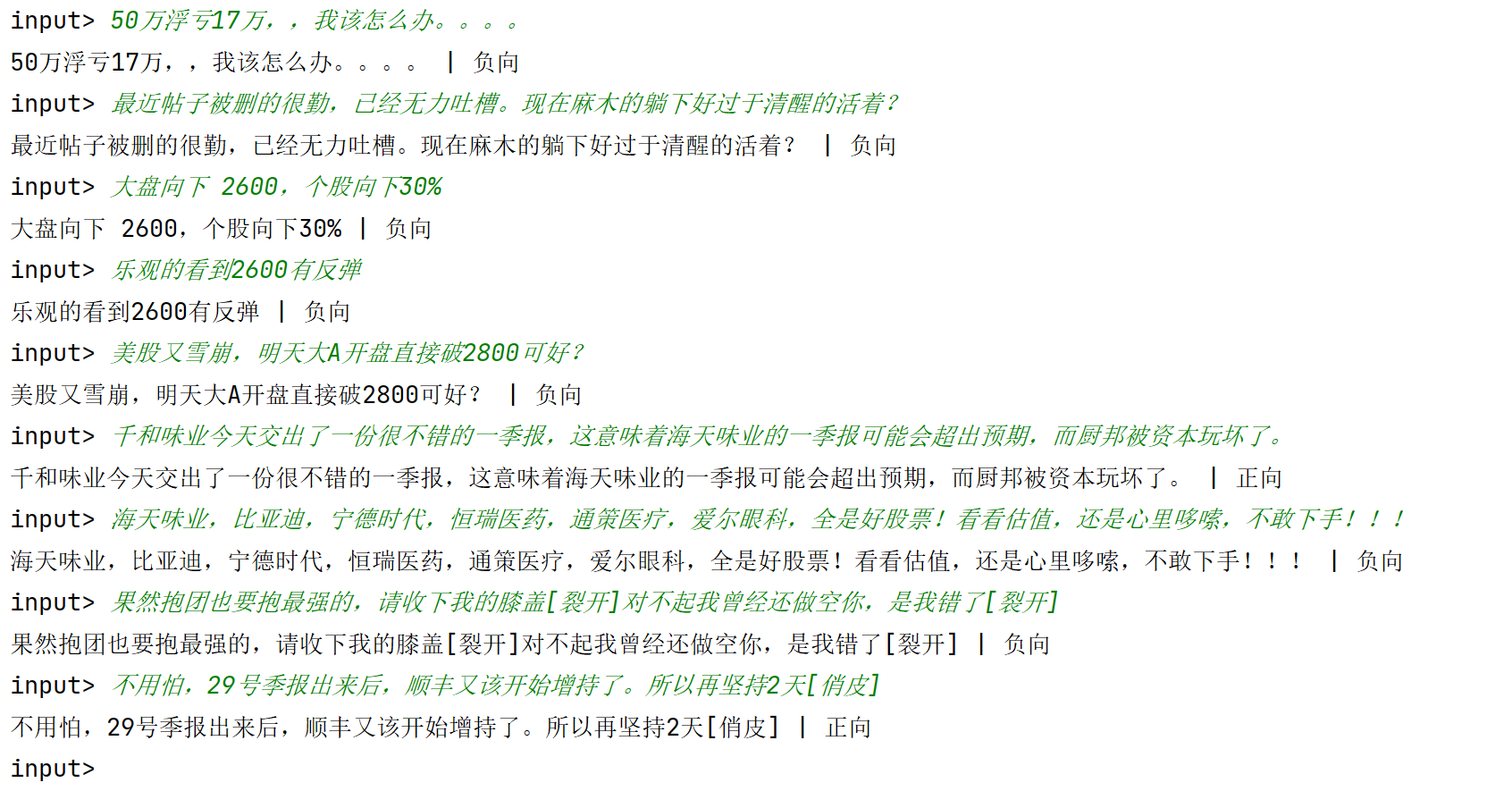### 七、模型的使用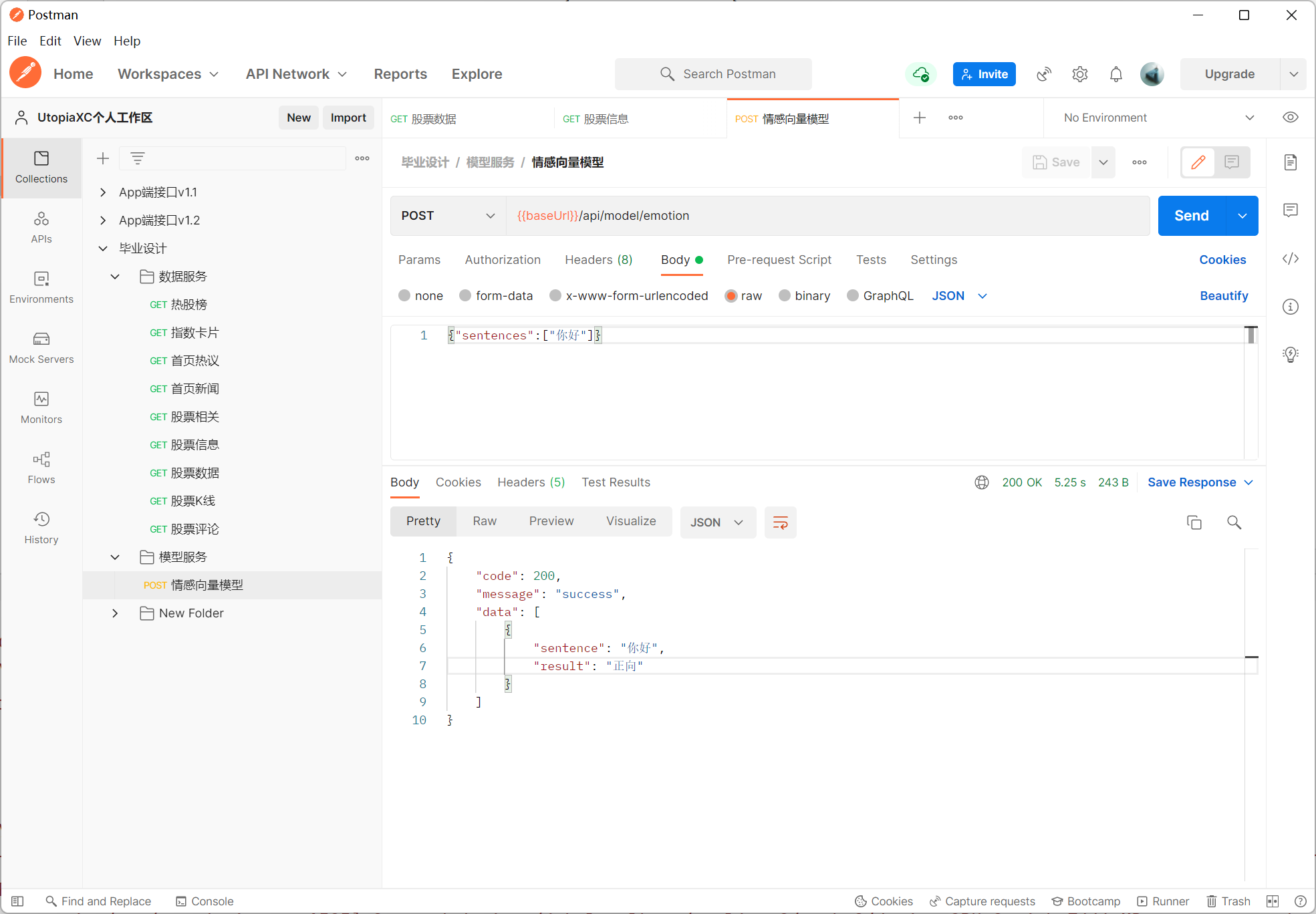2022-05-03 13:05
UtopiaXC